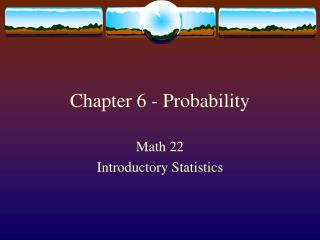DownloadDownload PresentationChapter 6 - Probability

# Chapter 6 - Probability

Télécharger la présentation## Chapter 6 - Probability

- - - - - - - - - - - - - - - - - - - - - - - - - - - E N D - - - - - - - - - - - - - - - - - - - - - - - - - - -
##### Presentation Transcript

1. Chapter 6 - Probability Math 22 Introductory Statistics

2. Simulating Repeated Coin Tosses • Simulation with the TI – 83 • Empirical Probability (Observed Probability) – The probability of a specific event as it was observed in an experiment. • Theoretical Probability – The true probability of a specific event of interest. Often an unknown value estimated by an empirical probability.

3. Probability • Probability - A numerical value that is associated with some outcome and indicates how likely it is that the outcome will occur. • Experiment - The process of making an observation or taking a measurement. • Sample Space (S) - Listing of all possible outcome of an experiment. • Event - Subset of the sample space.

4. Probability of an Event The probability of an event A is the sum of the outcomes in A. We write it as P(A). P(event) = # of times that the event can occur total # of outcomes in the experiment

5. Assigning Probabilities to Individual Outcomes In assigning probabilities to the individual outcomes in a sample space, two conditions must be satisfied: • The probability of each outcome must be between 0 and 1, inclusive. • The probabilities of all outcomes in the sample space must sum to 1.

6. Calculating the Probability of an Event • Define the experiment and list the outcomes in the sample space. • Assign probabilities to the outcomes such that each is between 0 and 1. • List the outcomes of the event of concern. • Sum the probabilities of the outcomes that are in the event of concern.

7. Law of Large Numbers • As the number of times an experiment is repeated increases (as n gets larger), the value of the empirical probability will approach the value of the theoretical probability.

8. Odds and Compliment of an Event • Odds • Compliment of an Event – The probability of that event nothappening.

9. General Addition Rule • Let A and B be events then,

10. Conditional Probability • Conditional Probability - The probability of an event occurring given that another event has already occurred.

11. The Multiplication Law for Independent Events • Let A and B be two independent events then P(A and B)=P(A)P(B)